# Norton’s Theorem | Norton’s Equivalent Circuit

Want create site? Find Free WordPress Themes and plugins.

## Norton’s Theorem states that:

Definition of Nortons TheoremAny two-terminal electric network comprising resistances and voltage and/or current sources may be substituted with a single current source connected in a parallel configuration with a single resistance. The current source output is the short-circuit current at the terminals of a network, whereas the parallel resistance is the resistance between the terminals of a network when all the sources are set to zero.

Suppose we are given an arbitrary circuit containing any or all of the following elements: resistors, voltage sources, current sources (the source can be dependent as well as independent). Let us identify a pair of nodes, say node a and b, such that the circuit can be partitioned into two parts as shown in figure 1.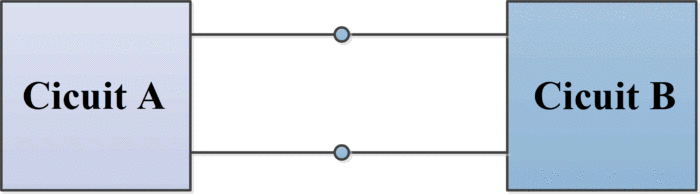Figure.1: circuit partitioned into two parts

Furthermore, suppose that circuit A contains no dependent source that is dependent on a variable in circuit B, and vice versa. Then, we can model circuit A by an appropriate independent current source, call it isc, which is connected in parallel with an appropriate resistance, call it RN. This parallel combination of a current source and a resistance is called Norton’s equivalent of circuit A. In other words, circuit A in figure 1 and the circuit in the shaded box in figure 2 have the same effect on circuit B. this result is known as Norton’s theorem.Figure.2: Norton’s Equivalent Circuit

Circuit B (which is often called a load) may consist of many circuit elements, a single element ( a load resistor), or no element.

## Norton’s Short Circuit Current

To obtain Norton’s equivalent of some circuit A, determine the short-circuit current isc by placing a short circuit between nodes a and b and then calculate the resulting current (directed from the terminal a to b through the short circuit).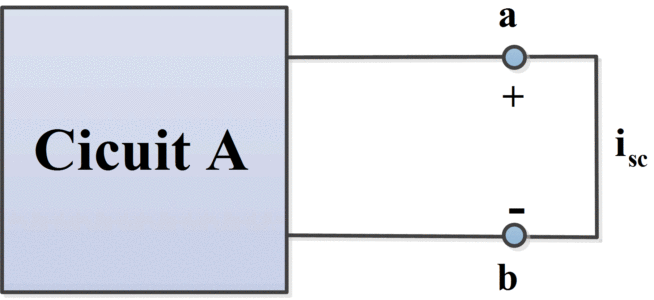Figure.3 (a): Determination of short-circuit current

## Norton’s Equivalent Resistance

There are several ways to find Norton’s equivalent resistant, which are given below:

1. To obtain the resistance RN– called Norton’s equivalent resistance of circuit A:
I. Remove circuit B from circuit A.
II. Set all independent sources in circuit A to zero. (A zero voltage source is equivalent to a short circuit, and zero current source is equivalent to an open circuit).
III. Determine the resistance between nodes a and b- this is RN– as shown in figure 3(b).Figure.3 (b): Determination of Norton’s Equivalent Resistance

2. Short-circuit the terminals a and b then find the short-circuit current Isc. The Norton’s equivalent resistance is given by

${{\text{R}}_{\text{N}}}\text{ = }{{\text{V}}_{\text{oc}}}\text{/}{{\text{I}}_{\text{sc}}}\text{ = }{{\text{V}}_{\text{th}}}\text{/}{{\text{I}}_{\text{sc}}}$

Whereas Voc or Vth can be found as was done for the Thevenin equivalent circuit.
3. When the source network has a ladder structure and contains no controlled (dependent) sources, RN is easily found by series-parallel reduction of the dead network. When the source network contains controlled sources, Norton’s resistance can be found using the method represented by figure 4.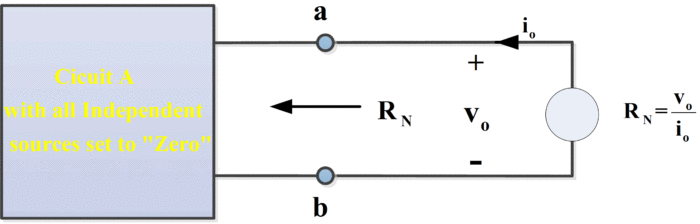Figure.4: Determination of Norton’s Equivalent Resistance

Here, the dead source network has been connected to an external test source. This test source may be any independent voltage or current source that establishes vo and it at the terminals. Since the dead network contains only resistors and controlled sources, and since RN equals the equivalent resistance of the dead network, the equivalent resistance theorem tells us that

${{\text{R}}_{\text{N}}}\text{=}{{\text{v}}_{\text{o}}}\text{/}{{\text{i}}_{\text{o}}}$

## Norton’s Theorem Solved Example

Find Norton’s Equivalent circuit of the following circuit.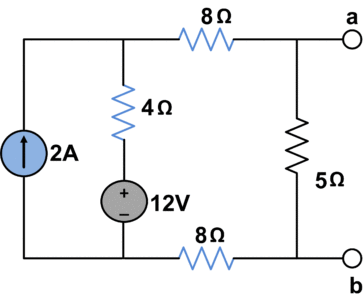Let’s find Norton Equivalent resistance RN

• Open circuit all current sources
• Short circuit all voltage sources

Now, we have the following circuit to calculate RN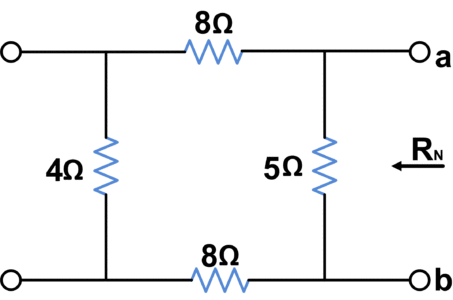${{R}_{N}}=\text{ }5||\text{ }\left( 8+4+8 \right)$

${{R}_{N}}=4\text{ }\Omega$

In order to find short-circuit current isc, we short circuit terminals a and b:Using loop analysis we have;

${{i}_{1}}=2A$

$20{{i}_{2}}-4{{i}_{1}}-12=0$

${{i}_{2}}=1A$

So;

${{I}_{N}}={{i}_{sc}}={{i}_{2}}=1A$

Hence we have following Norton’s equivalent circuit## Norton’s Theorem Solved Problem with Dependent Sources

We will find Isc and RN for the circuit shown in the following figure.In order to determine the short-circuit current, we’ll short-circuit terminals a and b as shown in the following figure and V2 = 0.Apply KCL to node 1:

$\begin{matrix} -0.003+\frac{{{V}_{1}}}{3000}+\frac{{{V}_{1}}}{2000}=0 & \cdots & (1) \\\end{matrix}$

Multiplying (1) by 6000 yields:

\begin{align} & 5{{V}_{1}}=\text{ }18 \\ & {{V}_{1}}=\text{ }3.6\text{ }V \\\end{align}

Now, calculating currents:

$i=\text{ }{{V}_{1}}/3000\text{ }=\text{ }3.6\text{ }V/3000\Omega =\text{ }0.0012\text{ }A$

${{V}_{CCVS}}=\text{ }2000i\text{ }=\text{ }2.4\text{ }V$

${{I}_{{{R}_{2}}}}=\text{ }{{V}_{1}}/{{R}_{2}}=1.8mA$

${{I}_{{{R}_{3}}}}=\text{ }{{V}_{CCVS}}/{{R}_{3}}=2.4mA$

Finally, IN would be

${{I}_{N}}={{I}_{{{R}_{2}}}}+\text{ }{{I}_{{{R}_{3}}}}~=\text{ }1.8mA+\text{ }2.4mA=\text{ }4.2mA$

In order to determine RN, deactivate the current source, a test voltage of 1 V is applied across terminals a and b as shown in the following figure. Vt = 1 V.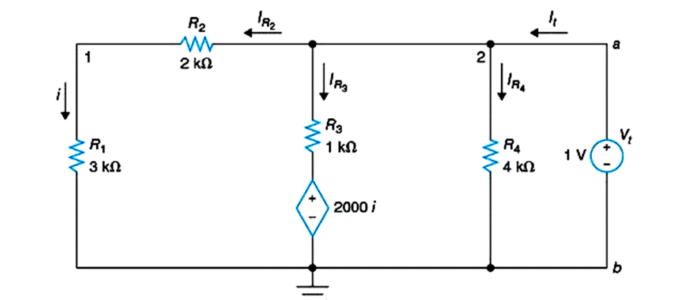${{I}_{{{R}_{2}}}}=i={{V}_{t}}/({{R}_{1}}+\text{ }{{R}_{2}})\text{ }=\text{ }1\text{ }V/5\text{ }k\Omega =0.2mA$

${{V}_{CCVS}}=\text{ }2000i\text{ }=\text{ }0.4\text{ }V$

${{I}_{{{R}_{3}}}}=\text{ }({{V}_{t}}\text{- }{{V}_{CCVS}})/{{R}_{3}}=0.6mA$

${{I}_{{{R}_{4}}}}={{V}_{t}}/{{R}_{4}}=0.25mA$

${{I}_{t}}={{I}_{{{R}_{2}}}}~+{{I}_{{{R}_{3}}}}~+{{I}_{{{R}_{4}}}}=\text{ }0.2mA+\text{ }0.6mA+\text{ }0.25mA=\text{ }1.05mA$

Noe, Norton’s equivalent resistance can be found as

${{R}_{N}}={{V}_{t}}/{{I}_{t}}=\text{ }952.381\Omega$

## Summary of Norton’s Theorem

Following steps can be carried out to obtain Norton equivalent circuit.

1. The Portion of circuit considered as load is removed
2. The short circuit current isc is calculated at terminals
3. To calculate RN:
• Short circuit all independent voltage sources
• Open circuit all independent current sources
1. Draw equivalent circuit, connect the load and calculate the load current.
Did you find apk for android? You can find new Free Android Games and apps.